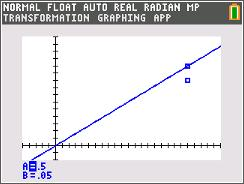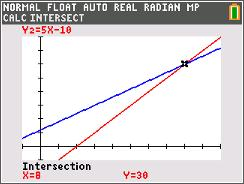# Activities

•• ##### Author45 Minutes

## Boats in Motion#### Activity Overview

Students explore the motion of a boat going up and down the river. They will be instructed to solve the resulting system of equations algebraically and graphically.

#### Key Steps

•In the first problem of this activity, students are introduced to the motion of two boats, one traveling downstream and the other traveling upstream. They will write and solve equations algebraically and graphically to find the speed of the boat in still water and the distance traveled.

•In the second part of the activity, students will explore the distance-time graph by changing the slope (or rate) of the equations. They will use the graph and algebraically solve to find the rate of the train and the rate of the person walking on the train.